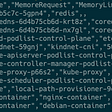# Gonum and Sparse package

First, let me answer this question, What is Gonum and Sparse package?
One of the most popular libraries in Go for scientific algorithms is the Gonum package. The Gonum package provides utilities that assist us in writing effective numerical algorithms using Go.

This library was created with performance in mind, the creators saw a problem with fighting vectorization in C, so they built this library in order to be able to manipulate vectors and matrices more easily in Go.

The Sparse library was built on top of the Gonum library in order to handle some of the normal sparse matrix operations that happen in machine learning and other parts of scientific computing.

This post is a brief part of “High Performance with Go” Which is edited a little by me.

## What is Vector?

A vector is a one-dimensional array that is often used for storing data. Go originally had a container/vector implementation, but this was removed on 18 October 2011, as slices were deemed more idiomatic for vector use in Go.
below, we have an easy way to portray a simple row vector using Go’s built-in functionality.

## What is Column Vector?

A column vector is an m x 1 matrix, also known as the transpose of a row vector. Matrix transposition is when a matrix is flipped over its diagonal, often denoted with a superscript T, Like below:

For implementing vector column we can use gonum package like below:

and the result of the above code is :

We can also do some neat vector manipulation with the Gonum package. For example, in the following code block, we can see how simple it is to double the values within a vector. We can add two vectors together using the AddVec function, thus creating a doubled vector. We also have the prettyPrintMatrix convenience function to make our matrix easier to read:

The gonum/mat package also gives us many other neat helper functions for vectors, including the following:

• Cap() gives you the capacity of the vector
• Len() gives you the number of columns within the vector
• IsZero() validates whether or not a vector is zero-sized
• MulVec() multiplies vectors a and b and serves the result
• AtVec() returns the value within the vector at a given position

The vector manipulation functions within the gonum/mat package help us to easily manipulate vectors into the datasets that we need.

## What is matrices?

Matrices are two-dimensional arrays, categorized by rows and columns. They are important in graphics manipulation and AI; namely, image recognition. Matrices are commonly used for graphics since the rows and columns that reside within a matrix can correspond to the row and column arrangement of pixels on a screen, as well as because we can have the matrix values correspond to a particular color.
Matrices are a good way to store a large amount of information in an efficient manner, but the manipulation of matrices is where the real value of matrices is derived from.
Matrices are usually denoted with an M × N naming scheme, where M is the number of rows in the matrix and N is the number of columns in the matrix.

The most commonly used matrix manipulation techniques are as follows:

At the end of this post, Let’s talk about a practical example of matrix multiplication so that we can tie our theoretical work into a workable example. Two separate electronic vendors are vying for your business to make widgets for your company. Vendor A and vendor B both design offerings for the widget and give you a parts list for what they’ll need. Both vendor A and vendor B use the same component supplier. In this example, we can use matrix multiplication to find out which vendor creates a less expensive widget. The parts list that each vendor gave you is as follows:

• Vendor A:
Resistors: 5
Transistors: 10
Capacitors: 2
• Vendor B:
Resistors: 8
Transistors: 6
Capacitors: 3

You know from the component’s supplier catalogue that the pricing for each of these components is as follows:

• Resistors cost: \$0.10
• Transistors cost: \$0.42
• Capacitors cost: \$0.37

and the result of the above code is :

--

--

--

I have a dream to have a spectacular garden

Love podcasts or audiobooks? Learn on the go with our new app.

## Recommended from Medium## VS Code + Docker + LaTeX Setup## Stop waiting weeks for downstream teams to root cause an issue## Monolithic vs Microservices Architecture## Why we built Clinic 🏥 w/ Botox 💉— a reverse proxy management platform## Deploy Django with Zappa + AWS## API DeOldify that turns monochrome images into colorI have a dream to have a spectacular garden

## Parsing the output of “kubectl get pods -A -o json” from a file## Connecting to Sockets in Go with A Retry Mechanism## Operating Systems: Virtualization V